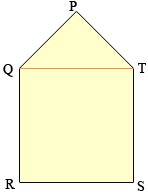From Mary, a secondary school student There is an (irregular) pentagon and it's made up of a square and a triangle. The triangle is directly on top of the square. The triangle is a right triangle. One of the angles is 45 degrees. one of the sides of the triangle is 4. what is the perimeter of the pentagon? Hi Mary, I drew a diagram of the situation you described. The angle at P is a right angle and one of the other two angles of the square measures 45 degrees, hence the third angle of the triangle also measures 45 degrees. Thus the sides PQ and TP are of equal length.You have said that one of the sides of the triangle has length 4 but you didn't say which side. This problem has two answers. If |PQ| = 4 then |TP| = 4 and you can use Pythagoras theorem to find |QT| and hence the perimeter of the pentagon. If |QT| = 4 then since |PT| = |TP| you can again use Pythagoras theorem this time to find |PQ| and hence the perimeter of the pentagon. Penny